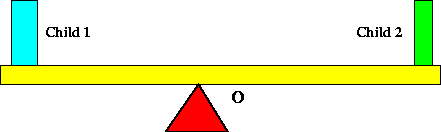Next: Moment of inertia Up: Torque Previous: Torque

## Static Equilibrium

The concept of torque is useful in determining whether or not a given object will be in static equilibrium. Consider two children, each of mass 30 kg, sitting on a teeter-totter as below.There are two general conditions for the teeter-totter to be stationary. The first is that the net force on it must vanish, since by Newton's second law (Force = mass x acceleration), a net force will cause an object to accelerate. In this situation this condition can be satisfied by requiring that total (downward) weight of the two children equals the (upward) force that the fulcrum exerts on the teeter-totter at O. However, this condition does not guarantee that the teeter-totter remain motionless, as it still might rotate about the fulcrum. To prevent this, one also requires that the net torque about the fulcrum must vanish. In this case there are two torques acting on the teeter-totter, one from each child, which act in opposite directions. Since the torque is the product of the force and distance from O, the force in this case being the weight of a child, and since the children have the same weight, we see that for the teeter-totter not to rotate that the children must sit at equal distance from O. We can also infer that if one child, for example, was twice as heavy as the other, that the heavier child would have to sit closer to O by a factor of 2 relative to the distance the lighter child is awayNext: Moment of inertia Up: Torque Previous: Torque
modtech@theory.uwinnipeg.ca
1999-09-29